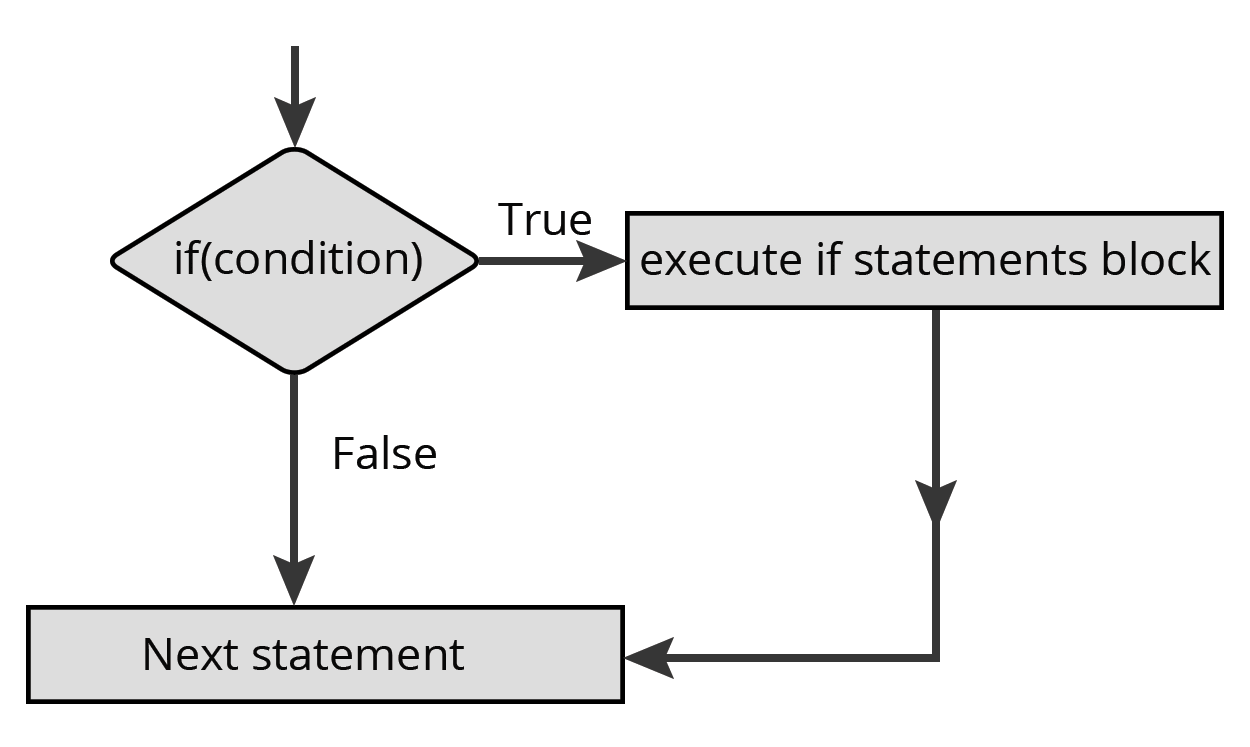Contents

• Python Tokens
• Variables and Assignments
• Simple Input/Output
• Data Types
• Mutable and Immutable Types
• Expressions
• If conditions
• Loops
• More Loops Lol

Python Tokens

The smallest unit in a program

Different Types of Tokens

• keyword
• Identifiers
• Literals
• Operators
• Punctuators

Keywords

Predefined words which has special meaning to the python interpreter. you can't use this to name your variables

Example Keywords

• False
• True
• None
• import
• pass
• break

there are more, but I am lazy to type it all :)

Identifiers

The names given by you is an identifier! example? the name of the variables and name of the functions.. there are more.. but I am lazy :)

• Variables should be non keyword with no space in between
# Invalid Variable
pass variable = "Hello"

# Valid Variable
variable = "World"
• made only with Letters, Numbers and Underscore (_)
# Invalid Variable
var-3 = "Hello"

# Valid Variable
var3 = "World"
# Invalid Variable
3var = "Hello"

# Valid Variable
var3 = "World"

Literals

Data items which has fixed/constant value.

String Literals

String, just imagine that its a word.

• Single line Strings
var = "This is a string"
var1 = 'String with single quotes'

• Multiline Strings
var = '''
hello
I am a multi line
string
'''

Numeric Literals

numeric literals? they are just numbers..

Integers

int they are just integers. 0, positive and negative numbers

Types

• Decimal - Base 10
• Octal - Base 8

Float

float they are numbers with decimal points 3.14159,10.32, etc

Boolean Literals

its just True and False nothing much tosay to be honest

None

None. its just none. if you asked something to python and python cannot find it, it returns None

Data Types

Lists

A group of comma seperated values of any datatypes between square brackets

lis = [1,2,3,4,5,6,7,8,9]
lis1 = ['q','w','e']
lis2 = [1,2,3,4,'1']

Tuples

A group of comma seperated values of any datatypes within parentheses.

tup = (1,2,3,4,5)
tup1 = ('q','w','e')
tup2 = (1,2,3,'e')

Sets

Mutable datatype like list but you can't have duplicates in it.

# Invalid Set
set1 = {1,1,3,4}

# Valid Set
set2 = {1,2,3,4}

Dictionaries

an unordered set of comma seperated key:value pairs within {}

dicto = {'a':1 ,'e':2 ,'i':3 ,'o':4}

If conditions

Plain If condition

var = True

if var:
print("Var is True")

You can also write it as.

var = True

if var == True:
print("var is True")

If-else statementsvar = True

if var == True:
print("Var is true")

else:
print("var is false")

If-elif statementsvar = "Hello"

if var == "Hello":
print("its hello")

elif var == "ok":
print("hi")

else:
print("hmm")